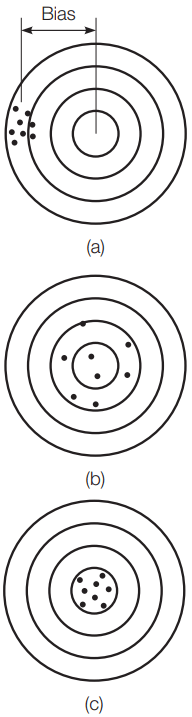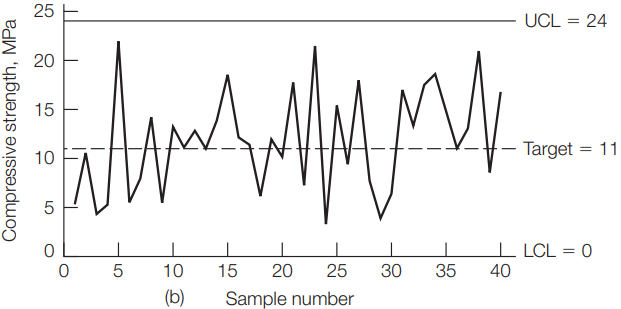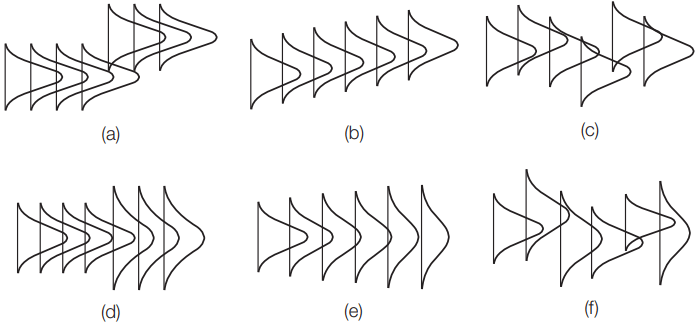# Variability of Materials

It is essential to understand that engineering materials are inherently variable. For example, steel properties vary depending on chemical composition and method of manufacture.

Concrete properties change depending on type and amount of cement, type of aggregate, air content, slump, method of curing, etc. The properties of asphalt concrete vary depending on the binder amount and type, aggregate properties and gradation, amount of compaction, and age.

Wood properties vary depending on the tree species, method of cut, and moisture content. Some materials are more homogeneous than others, depending on the nature of the material and the method of manufacturing. For example, the variability of the yield strength of one type of steel is less than the variability of the compressive strength of one batch of concrete.

Therefore, variability is an important parameter in defining the quality of civil engineering materials. When materials from a particular lot are tested, the observed variability is the cumulative effect of three types of variance: the inherent variability of the material, variance caused by the sampling method, and variance associated with the way the tests was conducted. Just as materials have an inherent variability, sampling procedures and test methods can produce variable results.

Frequently, statisticians call variance associated with sampling and testing error. However, this does not imply the sampling or testing was performed incorrectly.

When an incorrect procedure is identified, it is called a blunder. The goal of a sampling and testing program is to minimize sampling and testing variance so the true statistical features of the material can be identified. The concepts of precision and accuracy are fundamental to the understanding of variability.

Precision refers to the variability of repeat measurements under carefully controlled conditions. Accuracy is the conformity of results to the true value or the absence of bias. Bias is a tendency of an estimate to deviate in one direction from the true value. In other words, bias is a systematic error between a test value and the true value. A simple analogy to the relationship between precision and accuracy is the target shown in Figure 1.Figure 1. Exactness of measurements: (a) precise but not accurate, (b) accurate but not precise, and (c) precise and accurate.

When all shots are concentrated at one location away from the center, that indicates good precision and poor accuracy (biased) [Figure 1(a)]. When shots are scattered around the center, that indicates poor precision and good accuracy [Figure 1(b)]. Finally, good precision and good accuracy are obtained if all shots are concentrated close to the center [Figure 1(c)].

Many standardized test methods, such as those of the American Society for Testing and Materials (ASTM) and the American Association of State Highway and Transportation Officials (AASHTO), contain precision and bias statements. These statements provide the limits of acceptable test results variability. Laboratories are usually required to demonstrate testing competence and can be certified by the American Material Reference Laboratory (AMRL).

## Sampling

Typically, samples are taken from a lot or population, since it is not practical or possible to test the entire lot. By testing sufficient samples, it is possible to estimate the properties of the entire lot. In order for the samples to be valid they must be randomly selected. Random sampling requires that all elements of the population have an equal chance for selection.

Another important concept in sampling is that the sample must be representative of the entire lot. For example, when sampling a stockpile of aggregate, it is important to collect samples from the top, middle, and bottom of the pile and to combine them, since different locations within the pile are likely to have different aggregate sizes.

The sample size needed to quantify the characteristics of a population depends on the variability of the material properties and the confidence level required in the evaluation.

Statistical parameters describe the material properties. The mean and the standard deviation are two commonly used statistics. The arithmetic mean is simply the average of test results of all specimens tested. It is a measure of the central tendency of the population. The standard deviation is a measure of the dispersion or spread of the results.

The equations for the mean bar x and standard deviation s of a sample are

Where n is the sample size. The mean and standard deviation of random samples are estimates of the mean and standard deviation of the population, respectively. The standard deviation is an inverse measure of precision; a low standard deviation indicates good precision.

## Normal Distribution

The normal distribution is a symmetrical function around the mean, as shown in Figure 2. The normal distribution describes many populations that occur in nature, research, and industry, including material properties. The area under the curve between any two values represents the probability of occurrence of an event of interest.

Expressing the results in terms of mean and standard deviation, it is possible to determine the probabilities of an occurrence of an event. For example, the probability of occurrence of an event between the mean and {1 standard deviation is 68.3%, between the mean and {2 standard deviations is 95.5%, and between the mean and {3 standard deviations is 99.7%.

If a materials engineer tests 20 specimens of concrete and determines the average as 22 MPa and the standard deviation as 3 MPa, the statistics will show that 95.5% of the time the data will be in the range of 22 { (2 * 3), or 16 to 28 MPa.

If one wishes to estimate how close the mean of the data is to the mean of the population then the standard deviation of the mean, σ/(n)0.5 would be used. For the above example the standard deviation of the mean would be 3/(20)0.5 = 0.67 and 95.5% of the time the population mean would be in the range of 22 ; 2 * 0.67 or 20.66 to 23.34.

## Control Charts

Control charts have been used in manufacturing industry and construction applications to verify that a process is in control. It is important to note that control charts do not get or keep a process under control; they provide only a visual warning mechanism to identify when a contractor or material supplier should look for possible problems with the process. Control charts have many benefits, such as

• detect trouble early
• decrease variability
• establish process capability
• decrease inspection frequency
• provide a basis for altering specification limits
• provide a permanent record of quality
• provide basis for acceptance
• instill quality awareness

There are many types of control charts, the simplest of which plots individual results in chronological order. For example, Figure 3 shows a control chart of the compressive strength of concrete specimens tested at a ready-mix plant. The control chart can also show the specification tolerance limits so that the operator can identify when the test results are out of the specification requirements.

Although this type of control chart is useful, it is based on a sample size of one, and it therefore fails to consider variability within the sample. Statistical control charts can be developed, such as the control chart for means (X-bar chart) and the control chart for the ranges (R chart) in which the means or the ranges of the test results are chronologically plotted.Figure 4. Statistical control charts: (a) X-bar chart and(b) R chart. UCL indicates upper control limit; LCL indicates lowercontrol limit.

Figure 4(a) shows a control chart for the moving average of each three consecutive compressive strength tests. For example, the first point represents the mean of the first three tests, the second point represents the mean of tests two through four, and so on.

Figure 4(b) shows a control chart for the moving range of each three consecutive compressive strength tests. The key element in the use of statistical control charts is the proper designation of the control limits that are set for a given process.

These control limits are not necessarily the same as the tolerance or specification limits and can be set using probability functions. For example, the control chart for means relies on the fact that, for a normal distribution, essentially all of the values fall within ±3 standard deviations from the mean. Thus, control limits can be set between ±3 standard deviations from the mean. Warning limits to identify potential problems are sometimes set at ±2 standard deviations from the mean.

Observing the trend of means or ranges in statistical control charts can help eliminate production problems and reduce variability. Figure 5 shows possible trends of means and ranges in statistical control charts.Figure 5. Possible trends of means and ranges in statistical controlcharts: (a) sudden change in mean, (b) gradual change in mean, (c) irregularchange in mean, (d) sudden change in range, (e) gradual change in range, and(f) irregular change in mean and range.

Figure 5(a) shows sustained sudden shift in the mean. This could indicate a change of a material supplier during the project. A gradual change in the mean, as illustrated in Figure 5(b), could indicate a progressive change brought on by machine wear.

An irregular shift in the mean, as shown in Figure 5(c), may indicate that the operator is making continuous, but unnecessary, adjustments to the process settings. Figure 5(d) shows a sudden change in range, which could also indicate a change of a material supplier during the project.

Figure 5(e) shows a gradual increase in the range, which may indicate machine wear. Finally, Figure 5(f) shows an irregular shift in both mean and range, which indicates a flawed process.

## Experimental Error

When specimens are tested in the laboratory, inaccuracy could occur due to machine or human errors. For example, Figure 6 shows a stress–strain curve in which a toe region (AC) that does not represent a property of the material exists.